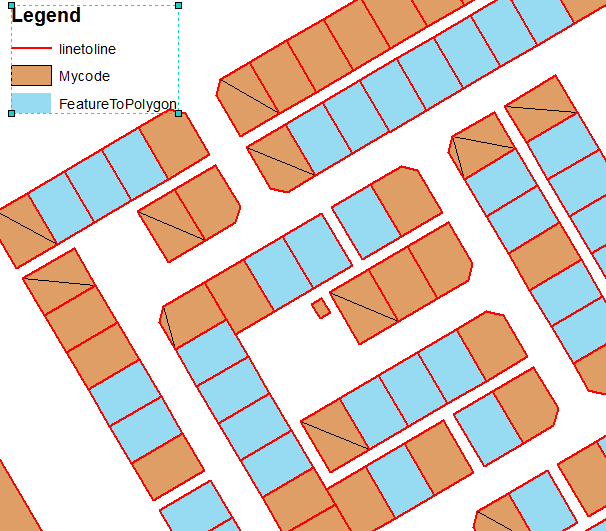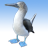# polyline to polygon (comparison tool and own code)

2326
11
05-22-2017 12:03 AMOccasional Contributor

I am trying to convert a ploy line feature class to polygon without using Arc info Advanced Licence.The code i am using runs fine and converts polyline to polygon. I have run the "tool feature to polygon" to make a comparison.My code is not converting all lines to polygon(See Screenshot).I have also attached my code.please guide me to get the same results.

Polyline :Red(to be converted)

Mycode:Brown

Feature to polygon: Blueimport arcpy

``````import arcpy
import os
from arcpy import env
env.overwriteOutput = True
starttime = time.time()
localtime = time.asctime( time.localtime(time.time()) )

# Create a value table that will hold the input feature classes for Merge

mxd=arcpy.mapping.MapDocument("CURRENT")
df = arcpy.mapping.ListDataFrames(mxd, "")

for lyr in arcpy.mapping.ListLayers(mxd,"",df):
if not lyr.isGroupLayer:
if "Export" in lyr.name :
break

desc = arcpy.Describe(lyr)
shapefieldname = desc.ShapeFieldName
spatialRef = arcpy.Describe(lyr).spatialReference
featureList = []

#array = arcpy.Array()
cursor = arcpy.da.InsertCursor(outPolys, ['SHAPE@'])
rows = arcpy.SearchCursor(lyr)
for row in rows:
# Create the geometry object
feat = row.getValue(shapefieldname)
#print "Feature %i: " % row.getValue(desc.OIDFieldName)
partnum = 0
part_list = []
# Step through each part of the feature
for part in feat:

polygonArray = arcpy.Array()
for pnt in feat.getPart(partnum):
if pnt:
part_list.append([pnt.X, pnt.Y])

partnum += 1
print partnum
print part_list
polygon = arcpy.Polygon(polygonArray)
featureList.append(polygon)

cursor.insertRow([polygon])
del cursor
endtime = time.time()
totaltime = endtime-starttime
print "\nScript took " + str(totaltime/60) + " minutes to run"

‍‍‍‍‍‍‍‍‍‍‍‍‍‍‍‍‍‍‍‍‍‍‍‍‍‍‍‍‍‍‍‍‍‍‍‍‍‍‍‍‍‍‍‍‍‍‍‍‍‍‍‍‍‍‍‍‍‍‍``````
Tags (1)
11 RepliesbyMVP Regular Contributor

I think your partnum counter on line 46 is indented one line too many.Occasional Contributor

Dear FC Basson,

partnum counter i hv only placed to check the polygon (coordinates ) only.I want to know what logic is behind feature to polygon tool?byMVP Regular Contributor

Yes, but you are incorrectly increasing the counter in the next indented for loop iterating the vertices and not in the part iteration.  So you will always just process the first (index 0) part and skip the rest.Occasional Contributor

Actually i just posted the code for creating polygon (i was using if statement ) and could not removed while posting.

I am displaying each polygon vertices and coordinates. The problem is that some line features have only two vertices (starting and ending) .My code is not converting them as it is not a polygon(we need at least three vertices).Please guide me to convert all lines as feature to polygon tool is converting.

import arcpy
import os
from arcpy import env
env.overwriteOutput = True
starttime = time.time()
localtime = time.asctime( time.localtime(time.time()) )

# Create a value table that will hold the input feature classes for Merge

mxd=arcpy.mapping.MapDocument("CURRENT")
df = arcpy.mapping.ListDataFrames(mxd, "")

for lyr in arcpy.mapping.ListLayers(mxd,"",df):
if not lyr.isGroupLayer:
if "Export" in lyr.name :
break

desc = arcpy.Describe(lyr)
shapefieldname = desc.ShapeFieldName
spatialRef = arcpy.Describe(lyr).spatialReference
featureList = []

#array = arcpy.Array()
cursor = arcpy.da.InsertCursor(outPolys, ['SHAPE@'])
rows = arcpy.SearchCursor(lyr)
for row in rows:
# Create the geometry object
feat = row.getValue(shapefieldname)
#print "Feature %i: " % row.getValue(desc.OIDFieldName)
partnum = 0
part_list = []
# Step through each part of the feature
for part in feat:

polygonArray = arcpy.Array()
for pnt in feat.getPart(partnum):
if pnt:
part_list.append([pnt.X, pnt.Y])

partnum += 1
print partnum
print part_list
polygon = arcpy.Polygon(polygonArray)
featureList.append(polygon)

cursor.insertRow([polygon])
del cursor
endtime = time.time()
totaltime = endtime-starttime
print "\nScript took " + str(totaltime/60) + " minutes to run"byMVP Regular Contributor

Could you please edit your post and use the Syntax Highlighter for your code? The indentation is cryptic as it currently is.Occasional Contributor
``````import arcpy
import os
from arcpy import env
env.overwriteOutput = True
starttime = time.time()
localtime = time.asctime( time.localtime(time.time()) )

# Create a value table that will hold the input feature classes for Merge

mxd=arcpy.mapping.MapDocument("CURRENT")
df = arcpy.mapping.ListDataFrames(mxd, "")

for lyr in arcpy.mapping.ListLayers(mxd,"",df):
if not lyr.isGroupLayer:
if "Export" in lyr.name :
break

desc = arcpy.Describe(lyr)
shapefieldname = desc.ShapeFieldName
spatialRef = arcpy.Describe(lyr).spatialReference
featureList = []

#array = arcpy.Array()
cursor = arcpy.da.InsertCursor(outPolys, ['SHAPE@'])
rows = arcpy.SearchCursor(lyr)
for row in rows:
# Create the geometry object
feat = row.getValue(shapefieldname)
#print "Feature %i: " % row.getValue(desc.OIDFieldName)
partnum = 0
part_list = []
# Step through each part of the feature
for part in feat:

polygonArray = arcpy.Array()
for pnt in feat.getPart(partnum):
if pnt:
part_list.append([pnt.X, pnt.Y])

partnum += 1
print partnum
print part_list
polygon = arcpy.Polygon(polygonArray)
featureList.append(polygon)

cursor.insertRow([polygon])
del cursor
endtime = time.time()
totaltime = endtime-starttime
print "\nScript took " + str(totaltime/60) + " minutes to run"

‍‍‍‍‍‍‍‍‍‍‍‍‍‍‍‍‍‍‍‍‍‍‍‍‍‍‍‍‍‍‍‍‍‍‍‍‍‍‍‍‍‍‍‍‍‍‍‍‍‍‍‍‍‍‍‍‍‍‍``````byMVP Esteemed Contributor

With an ArcGIS for Desktop Standard, you can use the Construct Polygons command on the Advanced Editing toolbar to create polygons from lines in ArcMap.

Think LocationOccasional Contributor

Dear Jayanta,

I know about these tools.My main concern is about the result comparison. I want to know the logic behind feature to polygon tool?there is something missing in my code.by
Occasional Contributor III

Put Simply, feature to polygon considers ALL INPUT FEATURES when creating shapes, and is a more complicated totally different algorithm than what you have begun to implement with your  "Feature by Feature" code.

You could look to dissolve the input lines, and then explode, as a pre-process, and then rewrite your own algorithym, but its probably a little complex to get good results!